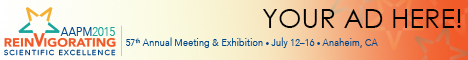# Program Information

## A Method for Predicting and Correcting the Dosimetric Effect of a Radiotherapy Treatment Couch in Actual Treatment PositionJ Duan

## Presentations

SU-E-T-417 Sunday 3:00PM - 6:00PM Room: Exhibit Hall

Purpose: Although radiation attenuation by the treatment couch can be included in the calculation of radiotherapy dose, difference between planned and actual treatment couch positions can generate significant dose discrepancies. We propose a method to predict and correct the dosimetric effect of the couch in actual treatment position.

Methods: The couch transmission factor, T, varies with beam angle, G, couch lateral position, x, and vertical position, y, i.e., T=T(x,y,G). If T(x,y,G) is known for a fixed couch vertical position y=h, the transmission of central-axis beam (CAX) T(x,y,G) can be obtained by T(x,y,G)=T(x⁺,h,G), where x⁺=x-(y-h)tan(G) and G is the angle between the beam and the vertical axis. Similarly, the transmission of any off-CAX point can be obtained using a similar formula. We measured CAX couch transmission at a fixed couch vertical position over the couch lateral motion range for all gantry angles by continuously scanning rotating arc beams. A 2D couch transmission correction matrix can thus be generated from T(x,h,G) for each treatment field for the actual couch position. By applying the transmission correction matrix to the planned field dose, the couch effect can be predicted and corrected. To verify this method, we measured couch transmission T(x, y=10cm, G=225°)(225°=IEC 135°) and compared to that obtained from equivalent T(x⁺, y=3cm, G=225°) over the range of lateral motion with a step size of 2 cm .

Results: The measured couch transmission factors T(x, y=10cm, G=225°) are in excellent agreement with those obtained from the equivalent T(x⁺, y=3cm, G=225°). The mean difference is 0.00406±0.00135.

Conclusion: The couch transmission correction matrix for any couch position and beam angle can be obtained from one set of scanning measurements at a fixed couch vertical position. The dosimetric effect of the treatment couch can be predicted and corrected by applying the couch transmission correction to the planned dose.

Contact Email: